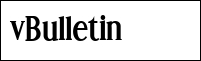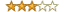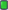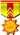ExetoolsMath Problem
 Register Forum Rules FAQ Calendar Mark Forums Read

 Notices https://forum.exetools.com This is the ONLY ONE domain that we use. Follow @exetools on Twitter and send me a message, I will choose whether to send the invitation code. Any password problems please mailto: 883600(at)qq(dot)com

#1KurapicaVIPJoin Date: Jun 2009 Location: Archives Posts: 165 Rept. Given: 20 Rept. Rcvd 129 Times in 37 Posts Thanks Given: 43 Thanks Rcvd at 266 Times in 63 PostsMath Problem

Hi

I need some help with a math problem, seems to be some kind of XORing encryption but not sure until now.

some people suggested it could be some kind of ECC encryption but there is no way to verify that, the code

that computes all this is not available, only input and output, I used guessing for the following results.

It's a function that takes the following 5 variables as input : R A B D E

and it returns P and Q , we need to calculate Q or Qy because finding one means we can calculate the other.

for each call, the RABDE variables change, but there seems to be a mathematical relation between the variables like this

Code:
//Static Values for all calls
F = 5716877567061631567
J = 42974360667485
G = 9438459873498734
H = 10598186474589962000

------------------------------------------------------
Static Mathematical relations even if input changes.
------------------------------------------------------
AxB = A ^ B  = 5716839026130626322

F  = AxB ^ J = 5716877567061631567
F =  Q ^ B ^ Qy
F =  P ^ A ^ Py

P =  A ^ Py ^ F
P =  B ^ Py ^ J

Q = P ^ A ^ Py ^ B ^ Qy
Q = A ^ Qy ^ J1
Q = F ^ B ^ Qy

Qy = Q ^ J ^ A
Qy = F ^ Q  ^ B

A = Qy ^ Q ^ J
B = Py ^ P ^ J

here are some correct solutions for input and output

Code:
Example 1 :

Qy = 8675903393861741358  Py = 1538555214519251343;
R = 811437463063792795;
A = 5529761018272509504;
B = 282568540822980946;

D = 3984046275;
E = 3690856581;

-------------------------------

P = 1635314993027775872;
Q = 3808514111295438899;

Code:
Example 2 :

Qy = 11516722568382942775
R = 1840606516305035782
A = 4805069320407829678
B = 1006910589681953724

D = 1670486575
E = 1432101603

-----------------------

P =  4837532237648236160
Q = 15959824880149022660

Code:
Example 3 :

Qy = 15812358381013069364
R = 8076138451608584365
A = 8027754194724583199
B = 2323300120116776973

D =          2402103439
E =          2581895634

------------------------

P =  5190706828787616960
Q = 12977299169235508342

I noticed that same input values can give a different value for P but the same value for Q all the time

for example, 5 calls to the function with same input gave the following result for P and Q

Code:
P = 7498808565226860864              Q = 5054277587748975737
P = 4741032886260565376              Q = 5054277587748975737
P = 2615148653128098944              Q = 5054277587748975737
P = 1353787373855115072              Q = 5054277587748975737
P = 7162932782392848192              Q = 5054277587748975737

so P and Py seem to be random every time and can be calculated like this

Code:
int main()
{

//different P but same Q every time

unsigned long long A =  5529761018272509504;
unsigned long long B =   282568540822980946;
unsigned long long F =  5716877567061631567;
unsigned long long Q = 0;
unsigned long long P = 0;
unsigned long long J = 42974360667485;

//P
unsigned long long Py = 4837532237648236160;  // make it random every time
P  = A ^ Py ^ F ;

return 0;
}

A proof of concept that randomizing P and Py can always produce the correct value for Q

Code:
#include <iostream>

using namespace std;

int main()
{
cout.precision(20);

//magic ‬

//dynamic variables
unsigned long long r = 811437463063792795;
unsigned long long a = 5529761018272509504;
unsigned long long b = 282568540822980946;

unsigned long long d = 3984046275;
unsigned long long e = 3690856581;

//static
unsigned long long f =  5716877567061631567;
unsigned long long g =     9438459873498734;
unsigned long long h = 10598186474589962000;

unsigned long long p = 0;
unsigned long long q = 3808514111295438899; // This is the correct value but it's still to be calculated later.

unsigned long long Py = 0;
unsigned long long Qy = 8675903393861741358; // This is the correct value but it's still to be calculated later.

unsigned long long j1 =       42974360667485;

//P
Py = 48448236160; //1538555214519251343; //?make it random every time ?
p  = a ^ Py ^ f ;

cout << " f =  " << f  << " ? " << (  q ^ b ^ Qy  ) << endl;
cout << " f =  " << f  << " ? " << (  p ^ a ^ Py  ) << endl;
cout << endl;
cout << " q =  " << q  << " ? " << ( p ^ a ^ Py ^ b ^ Qy) << endl;
cout << " q = "  << q << " ? " << ( a ^ Qy ^ j1 ) << endl;
cout << " q = "  << q  << " ? " << ( f  ^ b ^ Qy ) << endl;
cout << endl;
cout << " a =  " << a  << " ? " << ( Qy ^ q ^ j1 ) << endl;
cout << endl;
cout << " Py = " << Py << " ? " << ( p ^ f ^ a ) << endl;
cout << endl;
cout << " Qy = " << Qy << " ? " << ( q ^ j1 ^ a ) << endl;
cout << " Qy = " << Qy << " ? " << ( f ^ q ^ b ) << endl;
cout << endl;
cout << " p = "  << p << " ? " << ( b ^ Py ^ j1 ) << endl;
cout << " p = "  << p << " ? " << ( a ^ Py ^ f ) << endl;
cout << endl;
cout << " b =  " << b  << " ? " << ( Py ^ p ^ j1 ) << endl;
return 0;
}
 The Following 2 Users Say Thank You to Kurapica For This Useful Post: niculaita (01-15-2022), sh3dow (01-21-2022)
#2
 chantsVIPJoin Date: Jul 2016 Posts: 631 Rept. Given: 20 Rept. Rcvd 41 Times in 25 Posts Thanks Given: 578 Thanks Rcvd at 937 Times in 429 PostsThe usual variable names for ECC points P and Q being points on a curve is a distraction. ECC involves modular arithmetic with addition, multiplication, inverses. Quite clear this is not ECC.

The good news about XOR is it is bitwise addition modulo 2. There are many nice properties. Used alone it cannot really be used to protect anything as it's linear mapping and easily reversible. x^x==0, x^0==x, x^~x==1, x^1==~x. The basic properties are sufficient to invert this or simplify it.
 The Following User Says Thank You to chants For This Useful Post: niculaita (01-16-2022)
#3KurapicaVIPJoin Date: Jun 2009 Location: Archives Posts: 165 Rept. Given: 20 Rept. Rcvd 129 Times in 37 Posts Thanks Given: 43 Thanks Rcvd at 266 Times in 63 Postsyes those names of variables were my idea, the code itself is not available to make sure it involves any ECC or other known functions.

still I can't find the relation with Q or Qy as explained.
#4GitOld GitJoin Date: Mar 2002 Location: Torino Posts: 1,105 Rept. Given: 221 Rept. Rcvd 265 Times in 157 Posts Thanks Given: 102 Thanks Rcvd at 218 Times in 124 PostsI've no idea if any of this helps, and you probably know them anyway, but...

using you example :

R = 0x0B42 CE12 CF5C 8C9B
A = 0x4CBD A859 22EF 6E40
B = 0x03EB E297 3C74 4952
D = 0xED77 B8C3
E = 0xDBFE 0085

F = 0x4F56 6DDB A11B A24F
G = 0x0021 883A AFFC 966E
H = 0x9314 5272 7148 BF10
J = 0x0000 2715 BF80 855D

Lets take all the XORs in hex for reference :

R^F = 0x4414 a3c9 6e47 2ed4
R^G = 0x9856 9c60 be14 338b
R^H = 0x9856 9c60 be14 338b
R^J = 0x0b42 e907 70dc 09c6

A^F = 0x03eb c582 83f4 cc0f
A^G = 0xdfa9 fa2b 53a7 d150
A^H = 0xdfa9 fa2b 53a7 d150
A^J = 0x4cbd 8f4c 9d6f eb1d

B^F = 0x4cbd 8f4c 9d6f eb1d
B^G = 0x90ff b0e5 4d3c f642
B^H = 0x90ff b0e5 4d3c f642
B^J = 0x03eb c582 83f4 cc0f

D^F = 0x4f56 6ddb 4c6c 1a8c
D^G = 0x9314 5272 9c3f 07d3
D^H = 0x9314 5272 9c3f 07d3
D^J = 0x0000 2715 52f7 3d9e

E^F = 0x4f56 6ddb 7ae5 a2ca
E^G = 0x9314 5272 aab6 bf95
E^H = 0x9314 5272 aab6 bf95
E^J = 0x0000 2715 647e 85d8

Again, no idea if they have any significance, but I noted the following relations, for this example anyway :

R^G = R^H
A^G = A^H
B^G = B^H
D^G = D^H
E^G = E^H
(D^G) & 0xFFFFFFFF00000000 = (E^G) & 0xFFFFFFFF00000000
(D^J) & 0xFFFFFFFF00000000 = (E^J) & 0xFFFFFFFF00000000
(A^J) & 0xFFFFFFFF00000000 = (B^F) & 0xFFFFFFFF00000000
(A^F) & 0xFFFFFFFF00000000 = (B^J) & 0xFFFFFFFF00000000
(D^F) & 0xFFFFFFFF00000000 = (E^F) & 0xFFFFFFFF00000000

Git

 Thread ToolsShow Printable VersionEmail this Page Display ModesLinear ModeSwitch to Hybrid ModeSwitch to Threaded ModePosting Rules You may not post new threads You may not post replies You may not post attachments You may not edit your posts BB code is On Smilies are On [IMG] code is Off HTML code is Off Forum Rules

All times are GMT +8. The time now is 21:54.

 Aaron's homepage - TopAlways Your Best Friend: Aaron, JMI, ahmadmansoor, ZeNiX
( 1998 - 2021 )